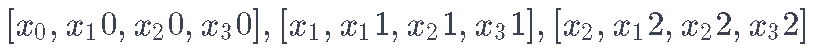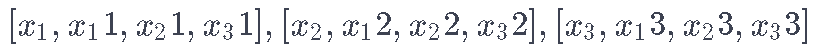# Python中TensorFlow的长短期记忆神经网络(LSTM)、指数移动平均法预测股票市场和可视化

## 本文探索Python中的长短期记忆（LSTM）网络，以及如何使用它们来进行股市预测。

LSTM模型很强大，特别是在保留长期记忆方面。在本文中，你将解决以下主题。

• 理解为什么你需要能够预测股票价格的变动。
• 下载数据 – 使用从雅虎财经收集的股市数据
• 分割训练-测试数据，并进行数据归一化
• 应用单步预测技术。
• 讨论LSTM模型。
• 用当前的数据预测和可视化未来的股票市场

## 为什么你需要时间序列模型？

LSTM神经网络架构和原理及其在Python中的预测应用

```!pip install tensorflow==1.14
# 可用的库
import numpy as np
import tensorflow as tf```

## 下载数据

• 开盘：当天的开盘股票价格
• 收盘价：当天的收盘股价
• 高点：数据中最高的股票价格
• 低点：当天的最低股价

# 获取数据

```    # 从URL中抓取数据
# 将日期、低点、高点、成交量、收盘价、开盘价存储到Pandas DataFrame中

#提取股票市场数据
df = pd.DataFrame(columns=\['Date', 'Low', 'High', 'Close', ' Open'\])
print('数据保存到：%s'%file\_to\_save)
# 如果数据已经存在，只需从CSV中加载即可
否则。
print('文件已经存在，从CSV中加载数据')

# 数据探索

```# 按日期对数据框架进行排序
df = df.sort_values('Date')

# 仔细检查结果

# R语言Keras用RNN、双向RNNs递归神经网络、LSTM分析预测温度时间序列、 IMDB电影评分情感

`plot(range(df.shape\[0\]),(df)/2.0)`

## 将数据分割成训练集和测试集

```train_data = mid\[:4000\]
test_data = mid\[4000:\]```

# 标准化数据

```将测试数据和训练数据相对于训练数据归一。

scaler = MinMaxScaler()```

# 提示：在选择窗口大小时，不要太小，因为当你进行窗口标准化时，会在每个窗口的最末端引入一个断点，因为每个窗口都是独立标准化的。

```# 用训练数据和平滑数据训练
window_size = 800
scaler.transform(train\_data\[di:di+window\_size,:\])```

`将数据重塑为[data_size]的形状。`

```# 重塑训练和测试数据
reshape(-1)

# 对测试数据进行标准化处理
scaler.transform(test_data).reshape(-1)```

```# 现在进行指数移动平均平滑处理
# 所以数据会比原来的锯齿状数据有一个更平滑的曲线

EMA = gamma\*train\[i\] + (1-gamma)\*EMA
train\[i\] = EMA```

# 标准平均

```    pred.append(np.mean(train\[idx-window_size:idx\]))
mse\_errors.append((std\_avg\[-1\]-train\[pred_idx\])**2)```
`MSE: 0.00418`

```plt.plot(std\_avg\_pred)

plt.legend(fontsize=18)
plt.show()```

# 指数移动平均线

```for idx in range(1,N):
mean = mean\*dec + (1.0-de)\*train\[idx-1\]
pred.append(mean)```
`MSE: 0.00003`
```plt.plot(mid_data)
plt.plot(pred)```

# 预测超过一步的未来股价

• 假设你得到的输出有以下公式

# LSTM简介：对股票走势进行远期预测

• 单元状态（ct）–这代表了单元的内部记忆，它同时存储了短期记忆和长期记忆
• _隐藏状态_（ht）–这是根据当前输入、以前的_隐藏状态_和当前的单元输入计算出来的输出状态信息，你最终会用它来预测未来的股市价格。此外，隐藏状态可以决定只检索存储在单元状态中的短期或长期或两种类型的记忆来进行下一次预测。
• 输入门（it）–决定当前输入的信息有多少流向单元状态
• 遗忘门（ft）–决定有多少来自当前输入和前一个单元状态的信息流入当前单元状态
• 输出门（ot）–决定多少信息从当前单元状态流入隐藏状态，因此，如果需要，LSTM可以只挑选长期记忆或短期记忆和长期记忆。

TensorFlow为实现时间序列模型提供了一个不错的API（称为RNN API）。

# 数据生成器

• 输入数据
•• 输出数据:
•# 数据增强(_Data_ _Augmentation_)

• x\_t+1,x\_t+2,…,xt+N不会彼此相距很远。

# 定义超参数

```D = 1 # 数据的维度。因为你的数据是一维的，所以是1
unrollings = 50 # 未来的时间步数。
batch_size = 500 # 一个批次中的样本数
num_nodes = \[200,200,150\] # 我们所使用的深层LSTM堆栈中每一层的隐藏节点数量
n\_layers = len(num\_nodes) # 层的数量
dropout = 0.2 # 丢弃量```

# 定义输入和输出

```#输入数据
train\_inputs, train\_outputs = \[\],\[\]```

# 定义LSTM和回归层的参数

### 计算LSTM输出并将其传递到回归层以获得最终预测结果

```# 创建单元格状态和隐藏状态变量保持LSTM的状态

for li in range(n):
c.append(tf.Variable(tf.zeros(\[batch\_size, num\_nodes\[li\]\])))
h.append(tf.Variable(tf.zeros(\[batch\_size, num\_nodes\[li\]\])))

# 做几次张量转换，因为函数dynamic_rnn要求输出必须是一种特定的格式。```

# 损失计算和优化器

```# 在计算损失时，你需要注意准确的形式，因为你计算的是所有未滚动的步的损失
# 因此，取每个批的平均误差，并得到所有未滚动步的总和

range(n)\]):
for ui in range(nums):
loss += tf.mean(0.5*(splits\[ui\]-train\[ui\])**2)```

# 预测相关的计算

```# 为预测阶段保持LSTM状态
for li in range(n_layers):
sample\_c.append(tf.Variable(tf.zeros(\[1, num\_nodes\[li\]\]))
sample\_h.append(tf.Variable(tf.zeros(\[1, num\_nodes\[li\]\])))```

# 运行LSTM

• 在时间序列上定义一个测试起点集（test\_points\_seq）来评估模型。
• 对于每个训练轮数
• 对于训练数据的完整序列长度
• 通过迭代测试点之前的num_unrollings数据点来更新LSTM状态
• 连续进行n\_predict\_once步骤的预测，将之前的预测作为当前输入。
• 计算预测的n\_predict\_once点与这些时间戳的真实股票价格之间的MSE损失
• 展开一组num_unrollings的批次
• 用未滚动的批次训练神经网络
• 计算平均训练损失
• 对于测试集的每个起点

# 预测可视化

```best_epoch = 28 # 用得到最佳结果的epoch

# 绘制预测值随时间变化的情况
# 绘制低α值的旧预测和高α值的新预测

plt.plot(xval,yval)

# 预测你得到的最佳测试预测值
plt.plot(range(df.shap),mid_data)

plt.plot(xval,yval)```

# 总结

1. 股票价格/走势预测是一项极其困难的任务。我个人认为，不应该把任何一个股票预测模型视为理所当然，盲目地依赖它们。然而模型可能在大多数时候能够正确预测股票价格走势，但不总是如此。
2. 不要被外面那些显示预测曲线与真实股票价格完全重合的文章所迷惑。这可以用一个简单的平均技术来复制，在实践中它是没有用的。更明智的做法是预测股票价格的变动。
3. 该模型的超参数对你获得的结果非常敏感。因此，要做的一件非常好的事情是对超参数运行一些超参数优化技术（例如，网格搜索/随机搜索）。下面我列出了一些最关键的超参数
• 优化器的学习率
• 层数和每层的隐藏单元的数量
• 模型的类型。你可以尝试GRU/标准LSTM和评估性能差异。

Kaizong Ye拓端研究室（TRL）的研究员。

​非常感谢您阅读本文，如需帮助请联系我们！QQ在线咨询

15121130882

0571-63341498

## 关注有关新文章的微信公众号

This will close in 0 seconds